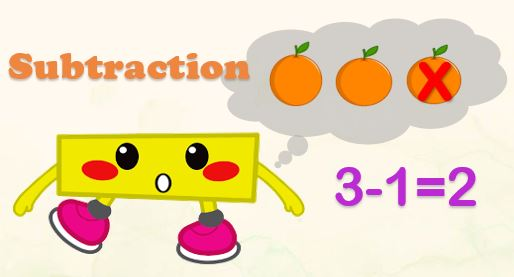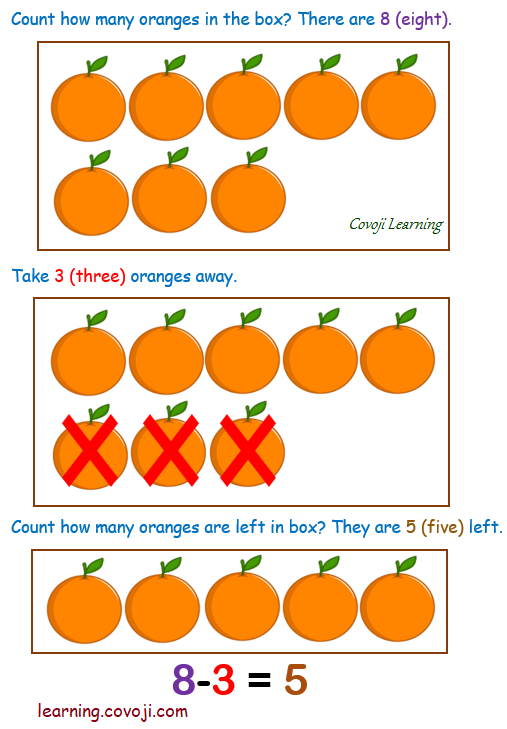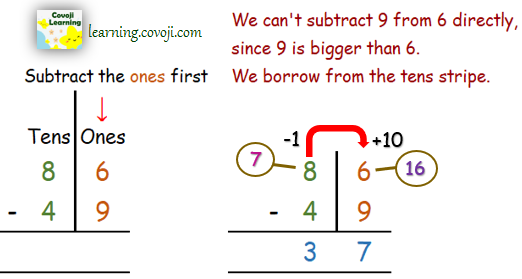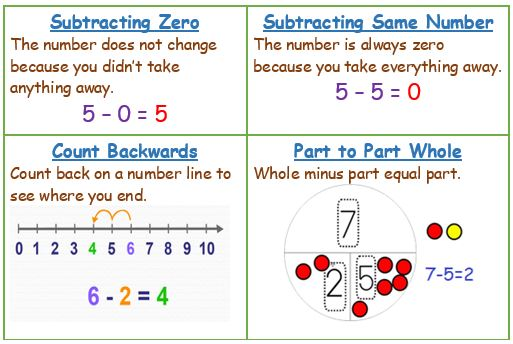Subtraction is taking one number away from another. Subtraction words are:

• minus
• less
• fewer
• difference

Understanding the concept of subtraction:Subtraction of large number

Regrouping, borrowing, or carrying over all refer to taking one set of tens and turning it into ten sets of ones. Or a set of hundreds and turning it into ten sets of tens and so on through the different place values of a given number. Let see how it works.Taking away in real life

Using every day activities to introducing them to subtraction concept. The use of chocolate is a great motivator! Count out chocolates bar and then say to your child: ‘How many will there be if I eat two?’ If your child gets confused, let them eat two chocolates and count the remaining chocolates.

Subtraction StrategiesCategories: Math Zone